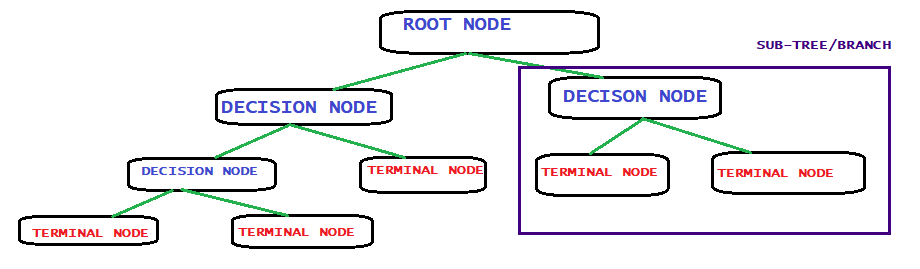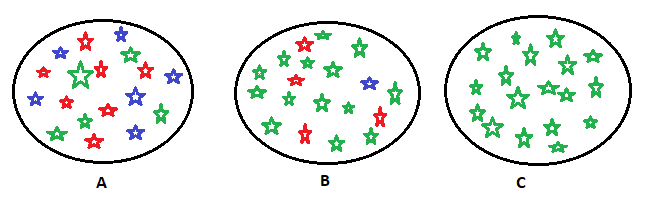# Most simple way to understand decision treePosted by

### Preface:

Decision tree(DT) and other tree-based methods are one of the most widely used supervised machine learning methods. These methods yield high accuracy at the same time they are easy to interpret. Unlike other machine learning methods, DT can map X and Y variables quite easily. Therefore, almost all of the tree-based model is simple to understand. A decision tree can be explained at any step exactly why the decision is made. As all the tree-based models are simple to interpret, it has relatively less processing time and uses fewer resources. Decision tree lays the foundations for more sophisticated tree bases models like the random forest, bagging, boosting.

In this blog, we shall discuss DT for both classifications and regression. And also we shall implement DT in python using some dummy dataset.

### CART models:

CART is the most widely used acronym for Classification and Regression Tree. All CART models represent a binary tree. The structure of the binary tree in algorithms is the same as the structure of CART models. The Decision tree belongs to the CART model. It is a supervised learning algorithm as it has a predefined target variable. We can use the DT for both classification and regression problems.

### Important terminologies:Root node: as the name suggests, this is the starting point of the tree.  it represents the whole data set. From this point, we shall divide the data set.

Splitting: it is a process partitioning dataset bases on some measures. Splitting increases the depth of the tree.

Decision node: it is a node from which more sub-nodes are created.

Leaf/terminal node: this is the end node. From this point, further division of the data set is not possible.

Pruning: is a method of truncating/chopping subnodes. Therefore, it is somewhat opposite to splitting. It reduced depth of the tree

Sub-tree/branch: it is a subset of the whole tree.

Parent node: it a node from which sub-nodes are generated.

Child node: it is node generated from the parent node.

### How the decision tree splits the dataset?

A dataset can have multiple types of features. In other words, we can say it is a heterogeneous data. Therefore, a dataset is most heterogeneous at the beginning process of the decision tree. Dataset splitting is a critical process as it directly affects the accuracy of the model. DT algorithm continues to split the dataset into smaller datasets until each sub-set of the data becomes homogeneous. This is the reason why tree-based methods outperform other ML algorithms.

As we know the decision tree algorithm can be used for both regression and classification problems. Therefore splitting of the node can is done based on the type of target variable. There are multiple ways to split the dataset. If the target variable is continuous, then Reduction in Variable method is used. And if the target variable is categorical type then, we can use Gini Impurity, Information Gain, Chi-Square. Few things to remember,  if we have a dataset which has c number of columns, then the dataset will be divided into c number of parts. Then each part of the dataset will be measured based on the splitting function used. Therefore, the subset with the most homogeneous result will be selected. That subset becomes the new node for a decision tree.

In the section below we shall dig deeper into each of these methods and see how we can use it.

### Splitting Methods 1: Reduction in Variance:

Reduction in Variance is used when there is a continuous target variable. In other words, if we have a regression problem in that case we can use Reduction in Variance. As we can infer from its name, it calculates variance in the dataset.X: represents the elements in the dataset.

µ: represents the mean of the dataset.

N: number of elements in the dataset.

How a reduction in variance works:

lets we have a dataset and it’s having only two features.  Question is: which feature to select for splitting? Well, we shall segregate the dataset with respect to(WRT) feature one and feature two. So, as a result of segregation, we are left with two subsets(node). In the next step, calculate the variance of each subset. The final step would be, select the node which has lower variance.

One point to be noted, As the DT algorithm splits dataset WRT each feature. Therefore, more the number of features, more the number of splits. DT will keep on splitting until the most homogeneous dataset is achieved.

### Splitting method 2: Gini Index/Co-efficient:

when we have a categorical target variable reduction in variance won’t work. In these cases, we use the Gini index.

Gini index is a statistical measure of distribution. Corrado Gini developed this measure, which is used to gauge wealth or income distribution among the population. The value of the Gini index ranges from 0 to 1. Where 0 represents perfect equality and 1 represents perfect inequality.

Dummy dataset:

Assume that we have a dataset where it has 3 features and 30 samples. Three features include gender, salary, income source employee/self-employed. The target variable is, someone will buy a property or not. Therefore, the target variable is categorical.

15 out of 30 people bought the property. There are 20 males, 10 females.  Out of 10 female,2 bought property(20%). Out of 20male, 13 bought property(65%). 12 people have less than 5000 salaries, out of the 5 bought property. 18 people have more than 5000 salaries, out of the 10 bought property. Out of 14 employed 6 bought property. Out of 16  self-employed,  9 bought the property.

Steps to calculate Gini:

The formula for calculating the Gini index is the sum of the square of probability for success and failure. The symbolic representation is (p^2+q^2). Where p is the probability of success and q is the probability of failure.

split on Gender, there are 10 females, 20% of them may buy property, so Gini of the female node would be:

2 female bought out of 10=0.2

8 female not bought out of 10=0.8

=(0.2)*(0.2)+(0.8)*(0.8)=0.68.

Similary, percentange of male bought property is 0.65 and percentange of male not bought =0.35

gini for male node: (0.65)*(0.65)+(0.35)*(0.35)=0.55

weighted gini for gender:

(0/30)*0.68+(20/30)*0.55 = 0.59

Split on income source:

employed: 6/14=0.428 bought the property, 8/14=0.57 did not buy a property.

Self-employed:9/16=0.56 bought the property, 7/16=0.43 did not but property.

Gini index for employed sub node: (0.428*0.428)+(0.57*0.57)=0.183

Gini index for self-employed sub node: ( 0.56*0.56)+(0.43*0.43)=0.313+0.189=0.502

Weighted gini on income source=(14/30)*0.51+(16/30)*0.51=51

Therefore, the Gini index is high if we consider gender as our splitting criterion.

### Splitting method 3: Chi-Square:

The chi-square static is primarily used for testing relationships between categorical variables. Basically there are two types of chi-square tests.

The first one determines how much a sample data is similar to a population. It is otherwise known as Goodness of Fit.

The second one compares two categorical variables and examines if they are related. This also reveals that whether the two categorical variables belong to the same distribution.

If the chi-square test is very small then there is a relationship between the two variables. In contrast, if the chi-square test is very high, then there is no relationship.E: it is the expected class of the child node.

O: it is the actual values for a class in a child node.

X2: it is the chi-square value for a class.

Steps to split a node using chi-square:

1. For each split, individually calculate the Chi-Square value of each child node by taking the sum of Chi-Square values for each class in a node
2. Calculate the Chi-Square value of each split as the sum of Chi-Square values for all the child nodes
3. Select the split with higher Chi-Square value
4. Until you achieve homogeneous nodes, repeat steps 1-3

### Splitting method 3: Information gain(IG):IG measures the degree of disorder in a dataset. If we consider the above three sets. We can describe figure C easily. In other words, set C is pure. We can easily classify the data points. In contrast to set C, if we consider set B, we need more complex mathematical equations to describe this. Set A is complete which is disordered. It does not convey any information to the user. Or we can say set A is impure.

The formula for calculating entropy is:P: probability of success.

Q:  probability of failure.

Steps to calculate entropy for the split:

1. Calculate the entropy of the parent node.
2. Calculate the entropy of child nodes and calculate the weighted mean of all the sub-nodes available.